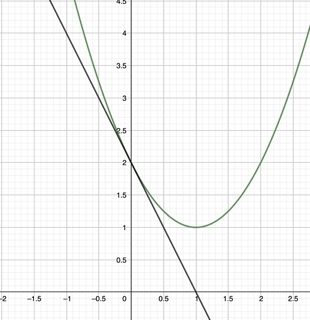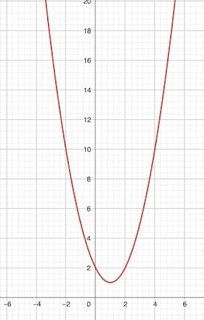# Rates of Change | Average & Instantaneous

Megan Krance, Betsy Chesnutt
• Author
Megan Krance

Megan has tutored in middle school level mathematics and high school level Algebra, Geometry, and Calculus for six years. They have a Bachelor's of Science Degree in Applied Mathematics from Robert Morris University in Moon Township, PA.

• Instructor
Betsy Chesnutt

Betsy has a Ph.D. in biomedical engineering from the University of Memphis, M.S. from the University of Virginia, and B.S. from Mississippi State University. She has over 10 years of experience developing STEM curriculum and teaching physics, engineering, and biology.

Learn how to calculate instantaneous rate of change and average rate of change from a graph. Compare instantaneous rate of change vs. average rate of change. Updated: 01/28/2022

Show

## Instantaneous Rate of Change vs. Average Rate of Change

Rate of change explains how quick something is changing. In mathematics, it is characterized as a rate of one quantity related to another quantity. The most common definition of the rate of change is the change in y divided by the change in x. The variable x is an independent variable, the variable y is a dependent variable. Rate of change can be positive, negative, or zero.

The instantaneous rate of change definition is the rate of change at a specific point in time. On a graph, the instantaneous rate of change is represented as a tangent line against the main function at one point.

Average rate of change is the rate of change of a function during a certain interval, for example, from 0 to 10, or from 2 to 7.

Instantaneous rate of change vs. Average rate of change boils down to whether the measurement is taken over an interval or at a specific instant in time.

### Example: Instantaneous and Average Rate of Change of Speed

A great example of rate of change in real life is the speed of a vehicle. When driving somewhere, the speed of the vehicle is constantly changing. When driving on a highway, the moments where someone speeds up to pass by someone is considered their instantaneous rate of change. Looking at someone's everyday driving habits and taking the average speed they drive is considered their average rate of change.

Another example are runners and their speed. A runner's top speed could be consisted their instantaneous rate of change. A runner's average speed over a course of races could be considered their average rate of change of speed.An error occurred trying to load this video.

Try refreshing the page, or contact customer support.

Coming up next: What is the Mean Value Theorem?

### You're on a roll. Keep up the good work!

Replay
Your next lesson will play in 10 seconds
• 0:03 What Is a Rate of Change?
• 1:11 Instantaneous vs Average Rates
• 2:02 Calculating from a Graph
• 3:10 Calculating with Calculus
• 4:42 When the Rates Are the Same
• 5:28 Lesson Summary
Save Save

Want to watch this again later?

Timeline
Autoplay
Autoplay
Speed Speed

## How to Calculate Instantaneous and Average Rate of Change

Using a graph or derivates are how to calculate the instantaneous and average rate of changes. The formula for calculating the average rate of change is:

{eq}(f(b) - f(a))/(b - a) {/eq}

The change in y values divided by the change in x values.

The formula for how to calculate the instantaneous rate of change is:

the limit as h approaches 0 of

{eq}(f(a +h) - f(a))/(a + h) {/eq}

In respect to x at a, the formula is now:

the limit as h approaches 0 of

{eq}(f(a +h) - f(a))/(h) {/eq}

This is otherwise known as the derivative of f(a). In conclusion, to find the instantaneous rate of change, find the derivate of the function at a certain time. This is using the equation of the curve to find the instantaneous rate of change.

Using a graph is another method of finding the rate of change. When using a graph to find instantaneous rate of change, instead of using the equation, use the actual curve. The instantaneous rate of change is a tangent line on a curve at a specific point. Then, find the slope of that tangent line.

### How to Find the Instantaneous Rate of Change from a Graph

Example:

Let the function be: {eq}f(x) = (x-1)^2 + 1 {/eq}

Graph it and draw a tangent line at the point {eq}(0,2) {/eq}How to find the instantaneous rate of change from a graph? Find the slope of the tangent line.

Solution: Finding the instantaneous rate of change at the point {eq}(0,2) {/eq}

To find the slope of the tangent line, take the point that is tangent to the curve and the point where the tangent line crosses the x-axis: {eq}(1,0) {/eq}

Slope = change in y values divided by change in x values

{eq}(2 - 0)/(0 - 1) = 2/-1 = -2 {/eq}

So, the instantaneous rate of change of this function is -2.

This is is similar to estimating the instantaneous rate of change. To estimate the instantaneous rate of change, draw a line on graph between two points near the point that is being asked for. Then, find the slope of that line.

### How to Find the Average Rate of Change from a Graph

Example: Find the average rate of change from a graph with the function: {eq}f(x) = (x-1)^2 + 1 {/eq} over the interval {eq}[-2,5] {/eq}

Solution:

Graph the function:{eq}= (f(5) - f(-2))/(5 - (-2)) {/eq}

{eq}= (17 - 10)/(5 - (-2)) {/eq}

{eq}= 7/7 {/eq}

{eq}= 1 {/eq}

The average rate of change from {eq}[-2,5] {/eq} is 1.

### Estimate the Instantaneous Rate of Change Using Derivatives

The derivative of a function {eq}dy/dx {/eq} represents the slope of {eq}y {/eq} with respect to {eq}x {/eq}.

The derivative is derived from the average rate of change formula:

{eq}(f(b) - f(a))/(b - a) {/eq}

Let {eq}b = a + h {/eq}

{eq}(f(a +h) - f(a))/(a + h - a) {/eq}

{eq}(f(a +h) - f(a))/(h) {/eq}

Using calculus, and taking the limit of that formula, gives the derivate of the function with respect to x. Therefore, providing the method to finding the instantaneous rate of change.

Example:

Let {eq}f(x) = x^3 + 2x^2 + 3x + 1 {/eq}. Find the instantaneous rate of change of the function at {eq}x = 2 {/eq}.

Solution:

Take the derivative of the function: {eq}f'(x) = 3x^2 + 4x + 3 {/eq}

Insert {eq}x = 2 {/eq}

{eq}f'(x) = (2)^2 + 4(2) + 3 {/eq}

{eq}f'(x) = 4 + 8 + 3 {/eq}

{eq}f'(x) = 15 {/eq}

The instantaneous rate of change of the function is 15.

To unlock this lesson you must be a Study.com Member.

#### How do you find the average rate of change in a graph?

When given an interval, locate the corresponding y values for each interval point on the graph. Then, divide the change in y values by the change in x values to find the average rate of change on a graph.

#### How do you find the instantaneous rate of change numerically?

You can find the instantaneous rate of change by taking the derivative of the function and inserting the instant of time being solved for. The derivate of the function is derived from the average rate of change formula because you are looking at a certain instant over the average interval.

#### How do you find the average rate of change?

Find the average rate of change by dividing the change in y, dependent variable, by the change in x, independent variable:

(f(b) - f(a))/(b - a)

On a graph, it is usually notated as "rise over run". Finding the average rate of change is similar to finding the slope of a line.

#### What does average rate of change represent graphically?

The average rate of change graphically represents how a function changed on average over an interval. It describes how the dependent variable, y, changed when the independent variable, x, changed.

### Register to view this lesson

Are you a student or a teacher?

Back

### Resources created by teachers for teachers

Over 30,000 video lessons & teaching resources‐all in one place.Video lessonsQuizzes & WorksheetsClassroom IntegrationLesson Plans

I would definitely recommend Study.com to my colleagues. It’s like a teacher waved a magic wand and did the work for me. I feel like it’s a lifeline.

Jennifer B.
TeacherCreate an account to start this course today
Used by over 30 million students worldwide